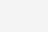# 使用Python – PCA分析进行金融数据分析

## 1.pandas的一个技巧

apply() 和applymap()是DataFrame数据类型的函数，map()是Series数据类型的函数。apply()的操作目标DataFrame的一列或许一行数据， applymap()是element-wise的，作用于每个DataFrame的每个数据。 map()也是element-wise的，对Series中的每个数据调用一次函数。

## 2.PCA分解德国DAX30指数

DAX30指数有三十个股票，听起来不多的样子，其实还是挺多的，我们很有必要对其进行主成分分析，然后找出最重要的几个股票。想必PCA的原理大家应该都是知道，说白了就是在一个回归中找到影响最大的那几个，当然，数学原理就涉及矩阵分解，什么SVD呀。

1. import pandas as pd
2. import pandas.io.data as web
3. import numpy as np
4. np.random.seed(1000)
5. import scipy.stats as scs
6. import statsmodels.api as sm
7. import matplotlib as mpl
8. import matplotlib.pyplot as plt
9. from sklearn.decomposition import KernelPCA#导入机器学习的PCA包
11.             ‘DB1.DE’,‘DBK.DE’,‘DPW.DE’,‘DTE.DE’,‘EOAN.DE’,‘FME.DE’,‘FRE.DE’,‘HEI.DE’,‘HEN3.DE’,
12.             ‘IFX.DE’,‘LHA.DE’,‘LIN.DE’,‘LXS.DE’,‘MRK.DE’,‘MUV2.DE’,‘RWE.DE’,‘SAP.DE’,‘SDF.DE’,
13.             ‘SIE.DE’,‘TKA.DE’,‘VOW3.DE’,‘^GDAXI’]#DAX30指数各个股票的代码以及德国30指数代码，共31个数据列
14. data = pd.DataFrame()
15. for sym in symbols:#获取数据
16.     data[sym] = web.DataReader(sym,data_source = ‘yahoo’)[‘Close’]
17. data = data.dropna()#丢弃缺失数据
18. dax = pd.DataFrame(data.pop(‘^GDAXI’))#将指数数据单独拿出来，采用pop在获取的时候已经从原来的地方删除了这一列数据了
19. scale_function = lambda x:(x-x.mean())/x.std()
20. pca = KernelPCA().fit(data.apply(scale_function))#这里用到了apply函数。做PCA前，我们要对数据做标准化
21. get_we = lambda x:x/x.sum()
22. print get_we(pca.lambdas_)[:10]

1. pca = KernelPCA(n_components = 1).fit(data.apply(scale_function))
2. dax[‘PCA_1’] =pca.transform(data)
3. dax.apply(scale_function).plot(figsize = (8,4))
4. pca = KernelPCA(n_components = 5).fit(data.apply(scale_function))
5. weights = get_we(pca.lambdas_)
6. dax[‘PCA_5’] =np.dot(pca.transform(data),weights)1. plt.figure(figsize = (8,4))
2. plt.scatter(dax[‘PCA_5’],dax[‘^GDAXI’],color = ‘r’)